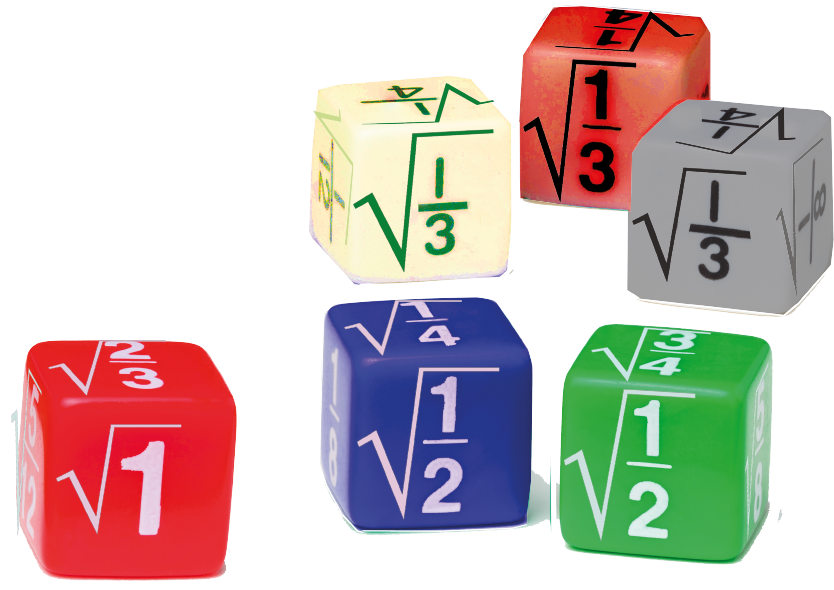# Rationalize the Denominator

Rationalize the Denominator
Go back to  'Irrational Numbers'

What is simplification?

Simplification in math is to write a number into its constituent factor, or to write a number in a format to help perform mathematical operations.

A rational fraction of the format $$\dfrac{a}{\sqrt b}$$ is simplified by removing the root symbol from the denominator by the process of rationalization.

Any of the arithmetic operations involving fractions can be performed conveniently only after rationalizing the denominatorIn this mini-lesson, we will explore the topic of rationalizing the denominator, by finding answers to questions like what is the meaning of rationalizing, how to rationalize the denominator using conjugates, and check the solved examples, interactive questions.

## What Is the Meaning of Rationalizing?

Rationalizing is the process of multiplying a surd with another similar surd, to result in a rational number.

The surd used to multiply is called the rationalizing factor (RF).

• To rationalize $$\sqrt x$$ we need another $$\sqrt x$$.  $\sqrt x \times \sqrt x = x$
• To rationalize $$a + \sqrt b$$ we need a rationalizing factor $$a - \sqrt b$$.  $(a + \sqrt b) \times (a - \sqrt b) = (a)^2 - (\sqrt b)^2 = a^2 - b$
• The rationalizing factor of $$2\sqrt3$$ is $$\sqrt3$$.  $2\sqrt3 \times \sqrt3 = 2\sqrt{3 \times 3} = 2 \times 3 = 6$

## How to Rationalize the Denominator Using Conjugates?

Before we learn how to rationalize a denominator, we need to know about conjugates.

A conjugate is a similar surd but with a different sign.

The conjugate of $$(\sqrt a + \sqrt b)$$ is $$(\sqrt a - \sqrt b )$$

In the process of rationalizing a denominator, the conjugate is the rationalizing factor.

The process of rationalizing the denominator with its conjugate is as follows.

\begin{align} \frac{1}{\sqrt a + \sqrt b} &= \frac{1}{\sqrt a + \sqrt b} \times \frac{\sqrt a - \sqrt b}{\sqrt a - \sqrt b } \\ &= \frac{\sqrt a - \sqrt b}{(\sqrt a)^2 - (\sqrt b)^2} \\ &= \frac{\sqrt a - \sqrt b}{a - b} \end{align}

Numbers and Number Systems
Numbers and Number Systems
grade 9 | Questions Set 2
Numbers and Number Systems